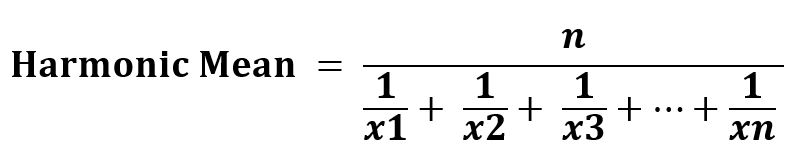Open In App

# sciPy stats.hmean() | Python

`scipy.stats.hmean(array, axis=0, dtype=None)` calculates the harmonic mean of the array elements along the specified axis of the array (list in python).

It’s formula –Parameters :
array: Input array or object having the elements to calculate the harmonic mean.
axis: Axis along which the mean is to be computed. By default axis = 0
dtype: Optional. It sets the type of returned element.

Returns : Harmonic mean of the array elements based on the set parameters.

Code #1:

 `# Harmonic Mean `` ` `from` `scipy.stats.mstats ``import` `hmean ``arr1 ``=` `hmean([``1``, ``3``, ``27``]) ``  ` `print``(``"Harmonic Mean is :"``, arr1) `

Output:

```Harmonic Mean is : 2.18918918919
```

Code #2: With multi-dimensional data

 `# Harmonic Mean `` ` `from` `scipy.stats.mstats ``import` `hmean``arr1 ``=` `[[``1``, ``3``, ``27``], ``        ``[``3``, ``4``, ``6``], ``        ``[``7``, ``6``, ``3``], ``        ``[``3``, ``6``, ``8``]] ``  ` `print``(``"\nHarmonic Mean is :"``, hmean(arr1)) `` ` `# using axis = 0``print``(``"\nHarmonic Mean is with default axis = 0 : \n"``, ``      ``hmean(arr1, axis ``=` `0``))`` ` `# using axis = 1``print``(``"\nHarmonic Mean is with default axis = 1 : \n"``, ``      ``hmean(arr1, axis ``=` `1``))  `

Output:

```Harmonic Mean is : [ 2.21052632  4.36363636  6.04195804]

Harmonic Mean is with default axis = 0 :
[ 2.21052632  4.36363636  6.04195804]

Harmonic Mean is with default axis = 1 :
[ 2.18918919  4.          4.66666667  4.8       ]
```